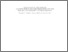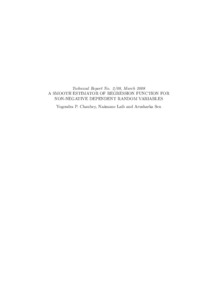Title:

# A Smooth Estimator of Regression Function for Non-Negative Dependent Random Variables

Chaubey, Yogendra P., Laib, Naâmane and Sen, Arusharka (2008) A Smooth Estimator of Regression Function for Non-Negative Dependent Random Variables. Technical Report. Concordia University. Department of Mathematics & Statistics, Montreal, Quebec.Preview Text (application/pdf) 2_08_Chaubey_Laib_Sen.pdf - Published Version 557kB

## Abstract

Commonly used kernel regression estimators may not provide admissible values of the regression function or its functionals at the boundaries, for regressions with restricted support. Any smoothing method will become less accurate near the boundary of the observation interval because fewer observations can be averaged, and thus variance or bias can be affected. Here, we adapt Chaubey et al. (2007)'s method of density estimation for nonnegative random variables to define a smooth estimator of the regression function. The estimator is based on a generalization of Hille's lemma and a perturbation idea. Its uniform consistency and asymptotic normality are obtained, for the sake of generality, under a stationary ergodic process assumption for the data . The asymptotic mean squared error is derived and the optimal value of
smoothing parameter is also discussed. Graphical illustration of the proposed estimator are provided on
simulated as well as real-life data.

Divisions: Concordia University > Faculty of Arts and Science > Mathematics and Statistics Monograph (Technical Report) Chaubey, Yogendra P. and Laib, Naâmane and Sen, Arusharka Department of Mathematics & Statistics. Technical Report No. 2/08 Concordia University. Department of Mathematics & Statistics Concordia University March 2008 Ergodic processes, Hille's Lemma, gamma density function, martin-gale difference, normality, prediction, regression function 6685 DIANE MICHAUD 03 Jun 2010 20:09 18 Jan 2018 17:29

## References:

Andrews, D.W.K., 1984. Non-strong mixing autoregressive processes. J. Appl. Probab. 21, 930-934.

Bagai, I. and Prakasa Rao, B.L.S. (1996). Kernel type density estimates for positive valued random variables. Sankhya. A57, 56-67.

Bosq, D., 1998. Nonparametric Statistics for Stochastic Processes. Lecture Note in Statistics. Springer, New York.

Bouezmarni, T. and Scaillet, O. (2005). Consistency of asymmetric kernel density estimators and smoothed histograms with application to income data. Econometric Theory 21, 390-412.

Chanda, K.C., 1974. Strong mixing of linear stochastic processes. J. Appl. Probab. 11, 401-408.

Chaubey, Y.P., Sen, A. and Sen, P.K. (2007). A new smooth density estimator for non-negative random variables. Submitted.

Chaubey, Y.P. and Sen, P.K. (1996). On smooth estimation of survival and density functions. Statist. Decisions 14, 1-22.

Chen, S.X. (1999). Beta kernel estimators for density functions. Comput. Statist. Data Anal. 31, 131-145.

Chernick, M.R., 1981. A limit theorem for the maximum of autoregressive processes with uniform marginal distributions. Ann. Probab. 9, 145-149.

Chow, Y.S. and Teicher, H. (1998). Probabilty Theory, 2nd ed. Springer, New York.

Feller, W. (1965). An Introduction to Probability Theory and its Applications, Vol II. New York: John Wiley and Sons.

Diaconis, P. and Freedman, D. (1999). Iterated random functions. SIAM Rev. 41, 41-76.

Engle, R.F., 1982. Autoregressive conditional heteroscedasticity with estimates of the variance of United
Kingdom inflation. Econometrica. 50, 987-1007.

Gasser, T. and Müller, H.G. (1979). Kernel estimation of regression functions. In: Smoothing Techniques for Curve Estimation, eds. Gasser and Rosenblatt. Heidelberg: Springer-Verlag.

Guégan, D., Ladoucette, S., 2001. Non-mixing properties of long memory processes. C. R. Acad. Sci. Paris, 333, 373-376.

Györfi, L. and Lugosi, G. (1992). Kernel density estimation from ergodic sample is not universally consistent. Comp. Stat. and Dta Analysis 14, 437-442.

Györfi, L., Morvai, G. and Yakowitz, S. (1998). Limits to consistent on line forecasting for ergodic time series. IEEE Transactions on Information Theory 44, 868-892.

Hall, P. and Wehrly, T.E. (1991). A geometrical method for removing edge e®ects from kernel-type nonparametric estimators. J. Amer. Statistical Assn., 86, 665-672.

Härdle, W. (1990). Applied nonparametric regression. Cambridge University Press.

Härdle, W. (1991). Smoothing Techniques with implementation in S. Springer Verlag.

Hall, P. and Heyde, C. (1980). Martingale Limit Theory and its Application. Academic Press. New York.

Hille, E. (1948). Functional Analysis and Semigroups. Am. Math. Colloq. Pub 31, New York.

Laïb, N., 2005. Kernel estimates of the mean and the volatility functions in a nonlinear autoregressive model with ARCH errors. J.S.P.I 134, 116-139.

Laïb, N. (1999). Exponential-type inequalities for martingale difference sequences: Application to non-
parametric regression estimation. Communication in Statistics, Theory and Methods, Series A, 28, 1565-1576.

Marron, J.S. and Ruppert, D. (1994). Transformations to reduce boundary bias in kernel density estimation. J. Roy. Statist. Soc., Ser B, 56, 653-671.

Müller, H.G. (1984). Boundary effects in nonparametric curve estimation models. In: COMPSTAT, 84-89, Physica Verlag.

Rice, J.A. (1984). Boundary modification for kernel regression. Communication in Statistics, Series A
13, 893-900.

Roussas, G.G. (1990). Nonparametric regression estimation under mixing conditions. Stochastic Processes. Appl 36, 107-116.

Ruppert, D. and Wand, M.P. (1992). Correcting for kurtosis in density estimation. Australian Journal of Statistics 34, 19-29.

Scaillet, O. (2004). Density esimation using inverse Gaussian and reciprocal inverse Gaussian kernels. Journal of Nonparametric Statistics 16, 217-226.

Silverman, B.W. (1986). Density estimation for statistics and data analysis. London: Chapman and Hall.

Tran, L.T. (1992). Kernel density estimation for linear processes. Stochastic Process. Appl 26, 281-296.

Tran, L.T. (1994). Density estimation for time series by histograms. Journal of Statistical Planing and inference 40, 61-79.

Tong, H., (1990). Non-linear time series: a dynamical system approach. Oxford University Press.

Wand, M.P., Marron, J.S. and Ruppert, D. (1991). Transformations in density estimation. Journal of
the American Statistical Association 86, 343-361.

Wu, W.B. and Shao X. (2004). Limit theorems for iterated random functions. J. Appl. Prob. 41, 425-436.

Wu, W.B, (2004). Nonlinear system theory: another look at dependence. Proc. Natl. Acad. Sci. USA 102, 40, 14150-14154 (electronic). 62M10

Wu, W.B., (2003). Nonparametric estimation for stationary processes. Technical Report, No. 536,
University of Chicago.

Zhang., S., Karunamuni, R.J. and Jones, M.C. (1999). An improved estimator of the density function at the boundary. J.Amer. Statistical Assn. 94, 1231-1241.
All items in Spectrum are protected by copyright, with all rights reserved. The use of items is governed by Spectrum's terms of access.

Repository Staff Only: item control pageResearch related to the current document (at the CORE website)
Back to top